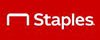# Casio FX-300ESPLS2-BU 16-Digit Scientific Calculator, Blue At \$9.99Deal Price.:\$9.99 M.R.P.: \$14.99 Discount: \$5  (33%OFF)## How to get the deal

• Add Product to the cart.
• Enter the shipping details.
• Make the final payment of your product.
• Enjoy the deal.

### Casio FX-300ESPLS2-BU 16-Digit Scientific Calculator, Blue

• Scientific calculator with Natural Textbook Display can display fractions, derivatives, integrals, sums, and other operations similar to their display in math textbooks
• Perfect choice for middle school through high school students learning general math, trigonometry, statistics, algebra I and II, pre-algebra, geometry, and physics
• Dimensions: 0.5″H x 3.13″W x 6.38″D
• Calculator is solar cell-powered
• 249 built-in math functions
• Features linear regression, quadratic regression, logarithmic regression, e-exponential regression, ab-exponential regression, power regression, and inverse regression
• Can transform a displayed decimal calculation result to a fraction or mixed number
0 0